##### Winter Tree
Currency conversion from USD to: [convert number=1350.00 from="usd" to="zar"] ZAR | [convert number=1350.00 from="usd" to="gbp"] GBP | [convert number=1350.00 from="usd" to="eur"] EUR     Medium: Oil and Gold Leaf on Canvas Size: 40cm by 540cm SOLD
\$ 1,350.00##### Snowy Owl
Currency conversion from USD to: [convert number=1400.00 from="usd" to="zar"] ZAR | [convert number=1400.00 from="usd" to="gbp"] GBP | [convert number=1400.00 from="usd" to="eur"] EUR     Medium: Oil and Gold Leaf on Canvas Size: 74cm by 50cm SOLD
\$ 1,400.00##### Crow’s Nest
Currency conversion from USD to: [convert number=1400.00 from="usd" to="zar"] ZAR | [convert number=1400.00 from="usd" to="gbp"] GBP | [convert number=1400.00 from="usd" to="eur"] EUR     Medium: Oil on Canvas Size: 40cm by 40cm SOLD
\$ 1,400.00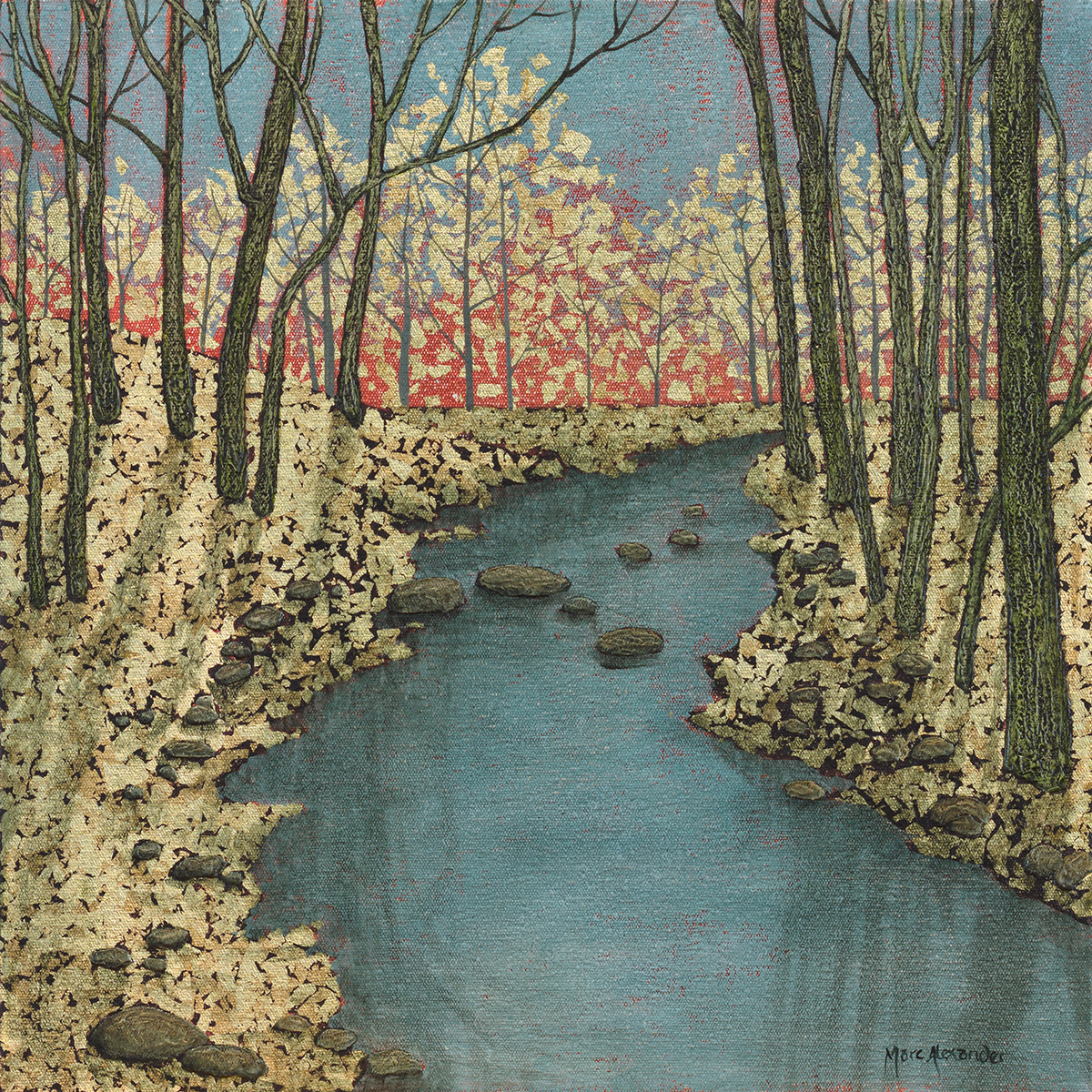##### Forest Stream I
Currency conversion from USD to: [convert number=1400.00 from="usd" to="zar"] ZAR | [convert number=1400.00 from="usd" to="gbp"] GBP | [convert number=1400.00 from="usd" to="eur"] EUR     Medium: Oil and Gold Leaf on Canvas Size: 40cm by 40cm SOLD
\$ 1,400.00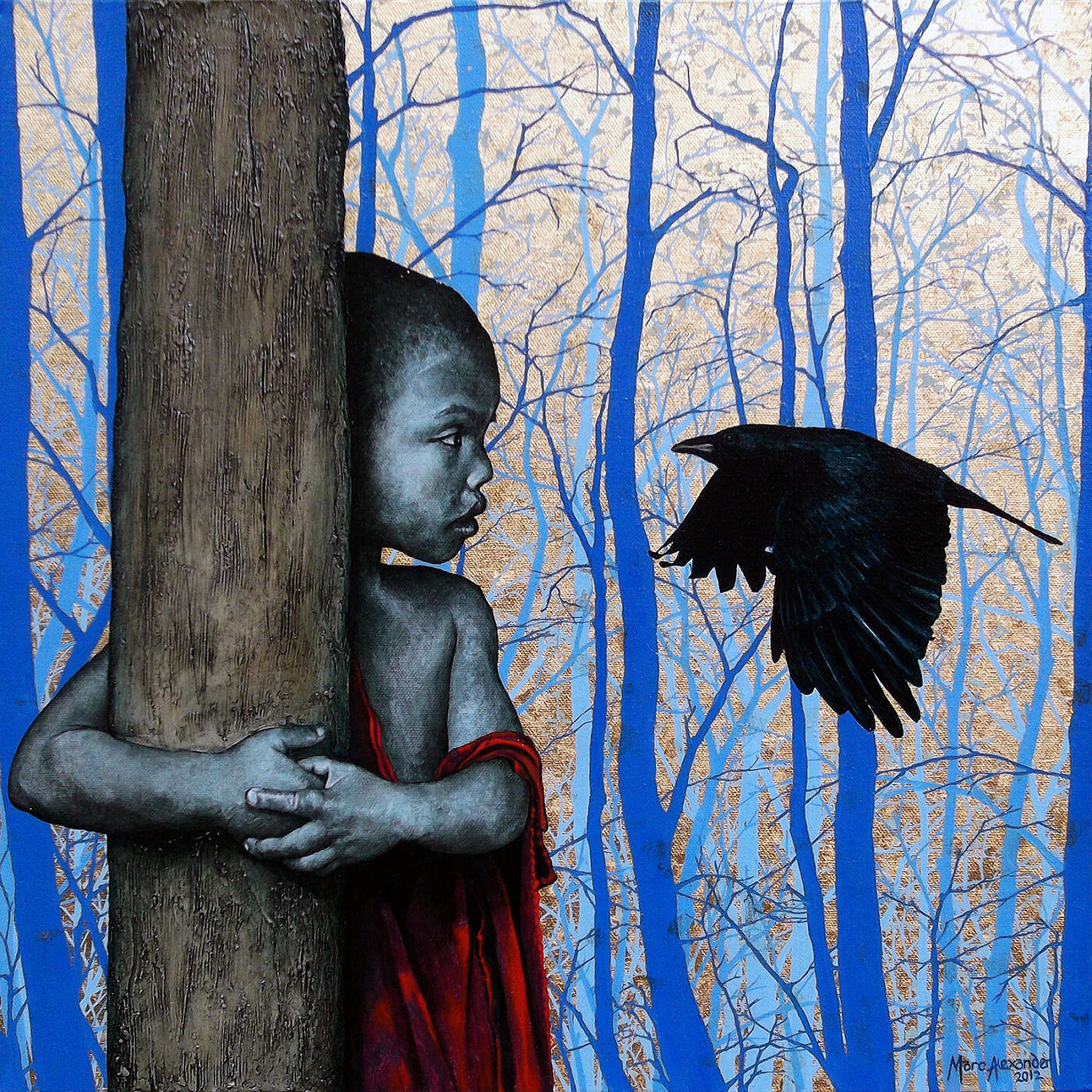##### Enchanted Forest I
Currency conversion from USD to: [convert number=1450.00 from="usd" to="zar"] ZAR | [convert number=1450.00 from="usd" to="gbp"] GBP | [convert number=1450.00 from="usd" to="eur"] EUR     Medium: Oil Gold Leaf on Canvas Size: 50cm by 50cm SOLD
\$ 1,450.00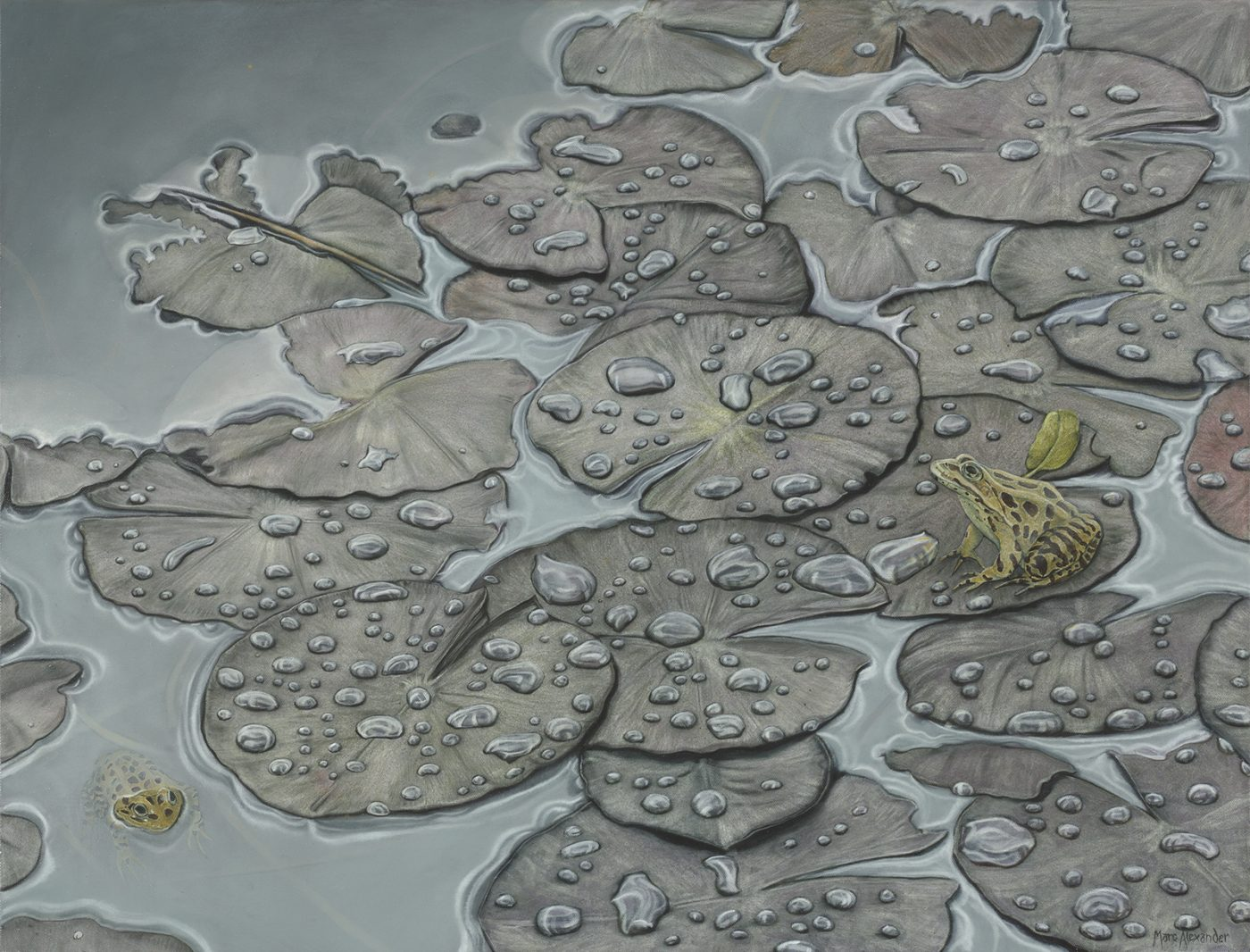Currency conversion from USD to: [convert number=1490.00 from="usd" to="zar"] ZAR | [convert number=1490.00 from="usd" to="gbp"] GBP | [convert number=1490.00 from="usd" to="eur"] EUR   Medium: Oil on Canvas Size: 68cm by 88cm SOLD
\$ 1,490.00
Sale!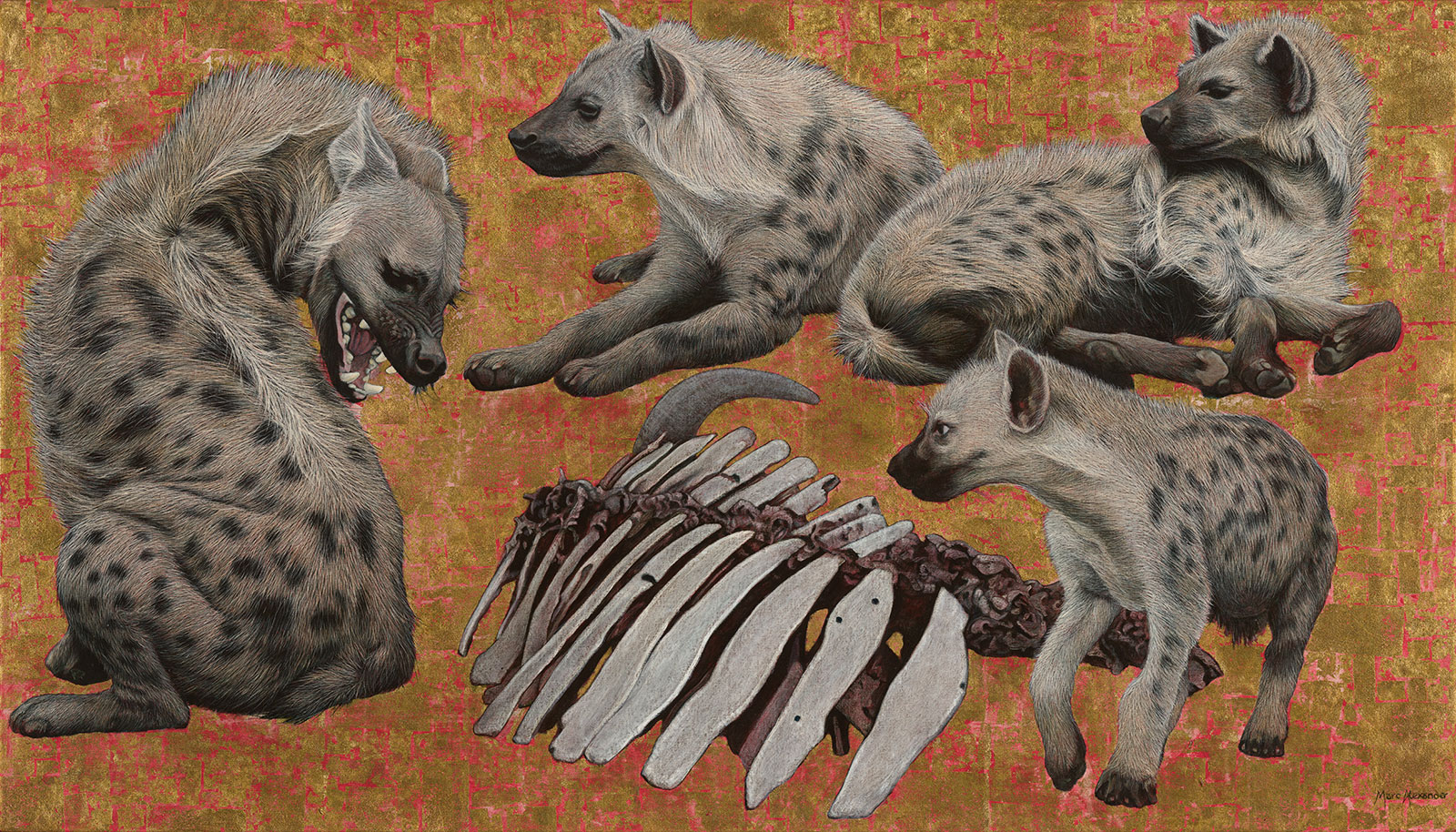##### Spotted Hyenas
Currency conversion from USD to: [convert number=1900.00 from="usd" to="zar"] ZAR | [convert number=1900.00 from="usd" to="gbp"] GBP | [convert number=1900.00 from="usd" to="eur"] EUR     Medium: Oil and Gold Leaf on Canvas Size: 80cm by 140cm
\$ 3,650.00 \$ 1,900.00
Sale!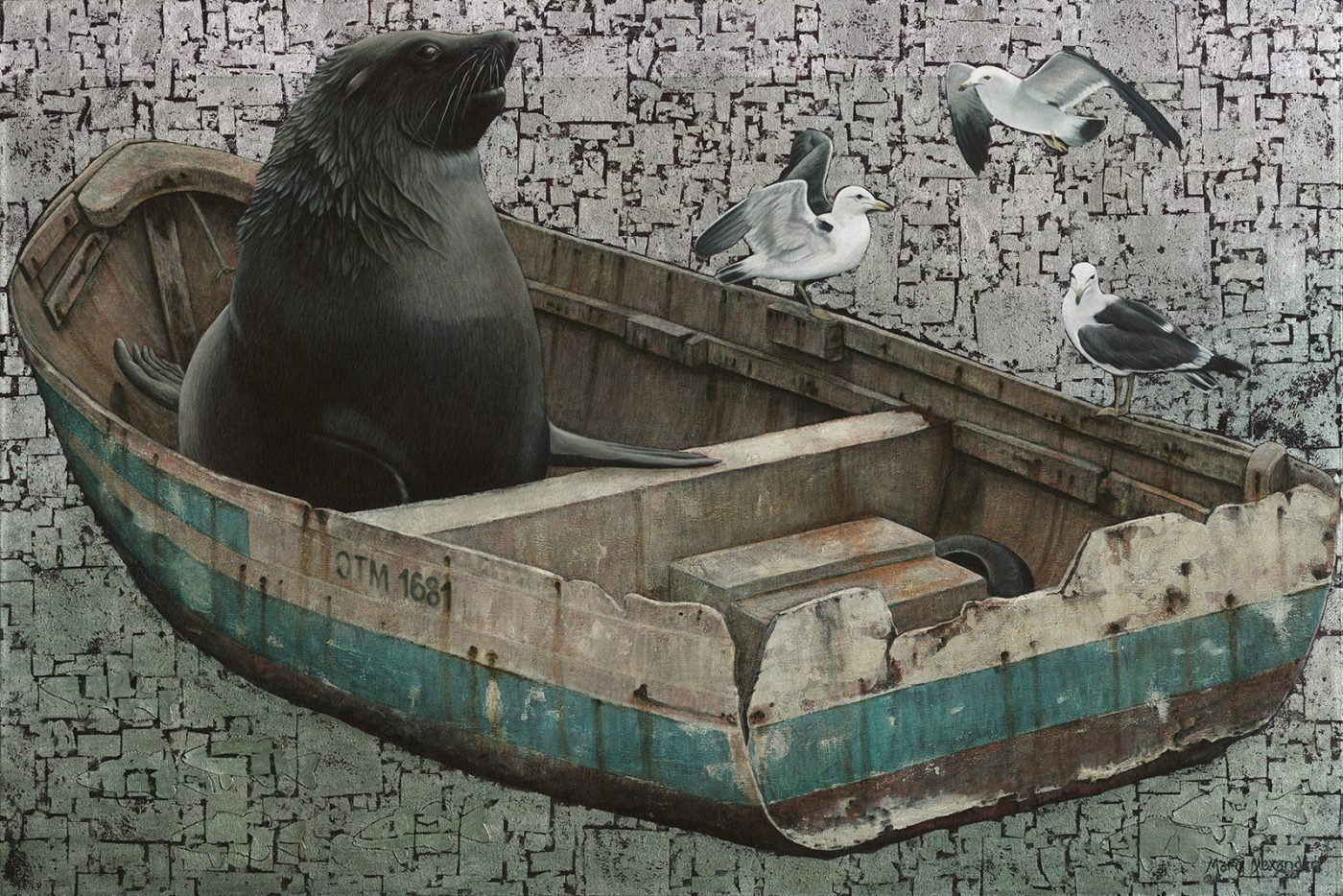##### Life of Arcto
Currency conversion from USD to: [convert number=2000.00 from="usd" to="zar"] ZAR | [convert number=2000.00 from="usd" to="gbp"] GBP | [convert number=2000.00 from="usd" to="eur"] EUR   Medium: Oil and Silver Leaf on Canvas Size: 100cm by 150cm
\$ 4,000.00 \$ 2,000.00##### Aloe I
Currency conversion from USD to: [convert number=2200.00 from="usd" to="zar"] ZAR | [convert number=2200.00 from="usd" to="gbp"] GBP | [convert number=2200.00 from="usd" to="eur"] EUR     Medium: Oil on Canvas Size: 80cm by 140cm SOLD
\$ 2,200.00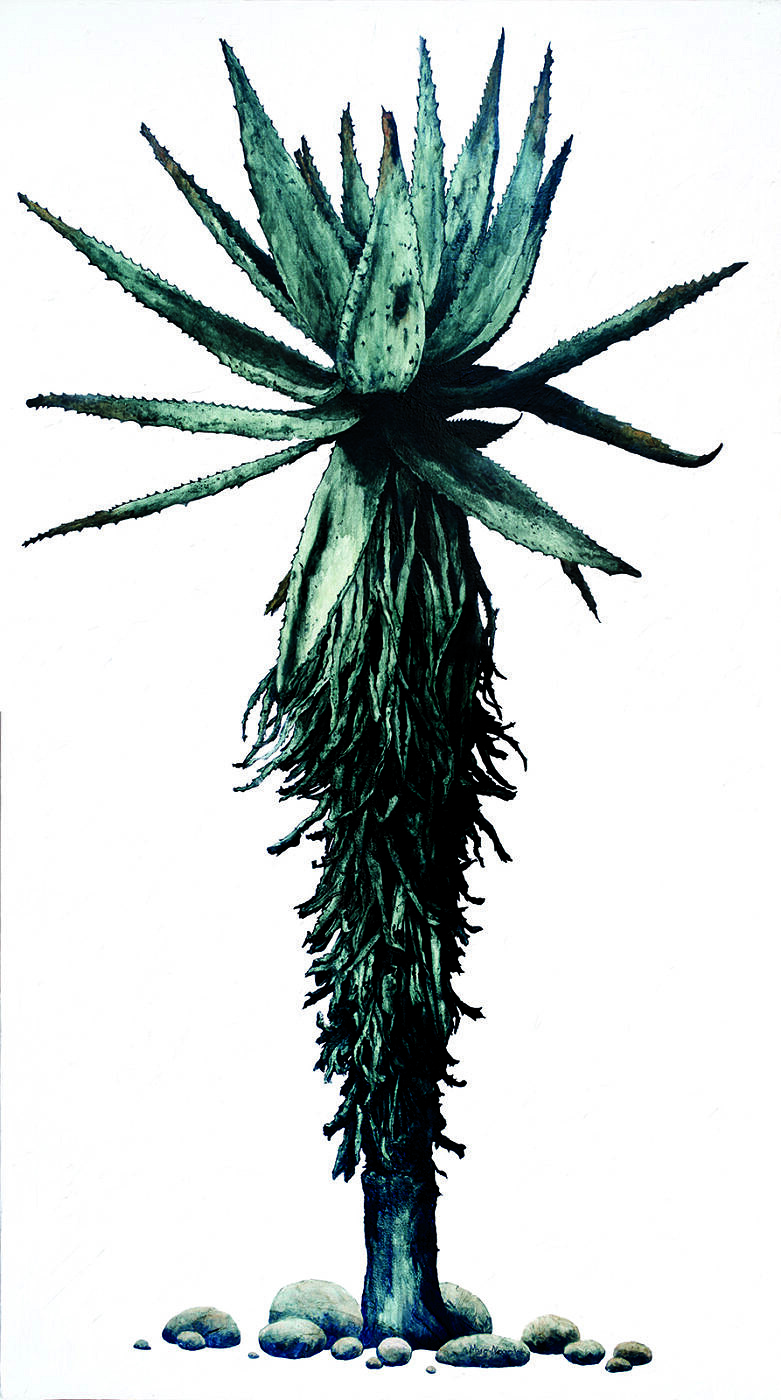##### Aloe III
Currency conversion from USD to: [convert number=2200.00 from="usd" to="zar"] ZAR | [convert number=2200.00 from="usd" to="gbp"] GBP | [convert number=2200.00 from="usd" to="eur"] EUR     Medium: Oil on Canvas Size: 80cm by 140cm SOLD
\$ 2,200.00
Sale!##### Enchanted Forest III
Currency conversion from USD to: [convert number=2200.00 from="usd" to="zar"] ZAR | [convert number=2200.00 from="usd" to="gbp"] GBP | [convert number=2200.00 from="usd" to="eur"] EUR     Medium: Oil and Gold Leaf on Canvas Size: 90cm by 120cm
\$ 3,950.00 \$ 2,200.00##### Himba Children
Currency conversion from USD to: [convert number=2200.00 from="usd" to="zar"] ZAR | [convert number=2200.00 from="usd" to="gbp"] GBP | [convert number=2200.00 from="usd" to="eur"] EUR     Medium: Oil and Gold Leaf on Canvas Size: 90cm by 120cm SOLD
\$ 2,200.00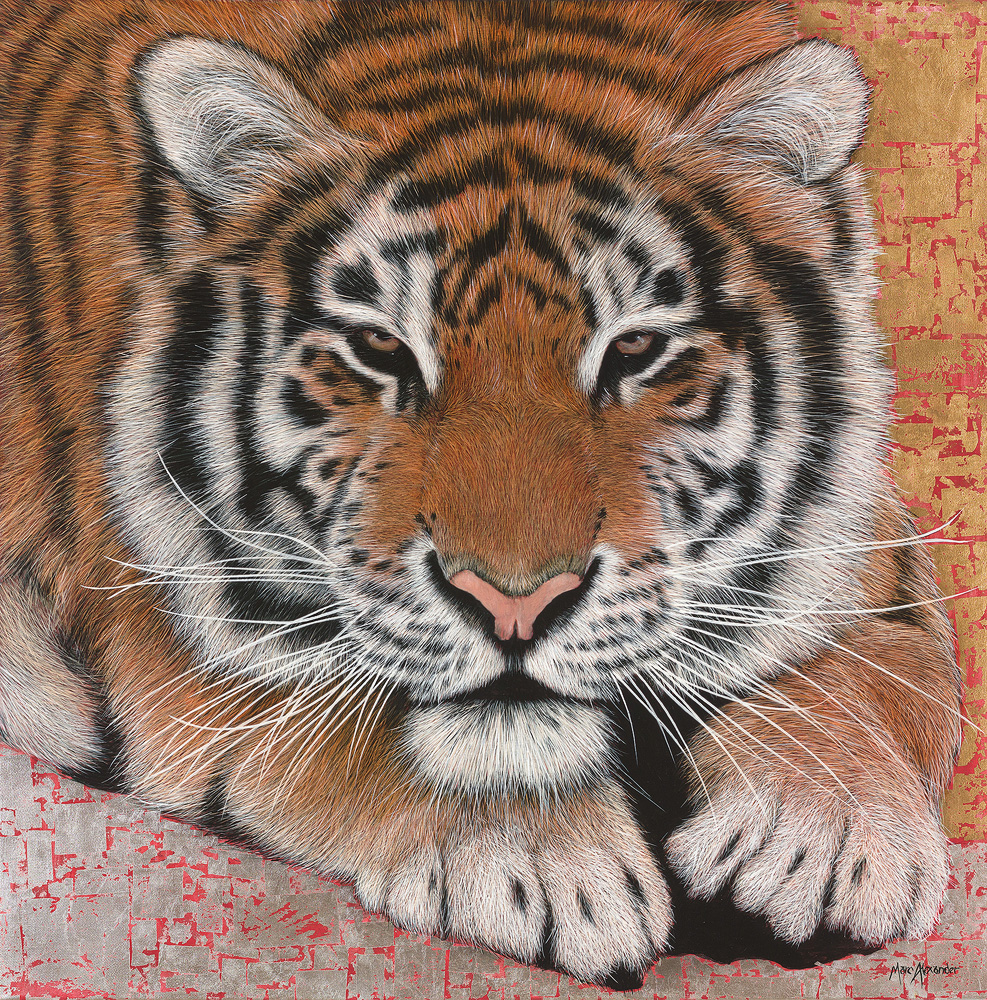##### Siberian Tiger
Currency conversion from USD to: [convert number=2250.00 from="usd" to="zar"] ZAR | [convert number=2250.00 from="usd" to="gbp"] GBP | [convert number=2250.00 from="usd" to="eur"] EUR     Medium: Oil and Silver leaf on Canvas Size: 90cm by 90cm SOLD
\$ 2,250.00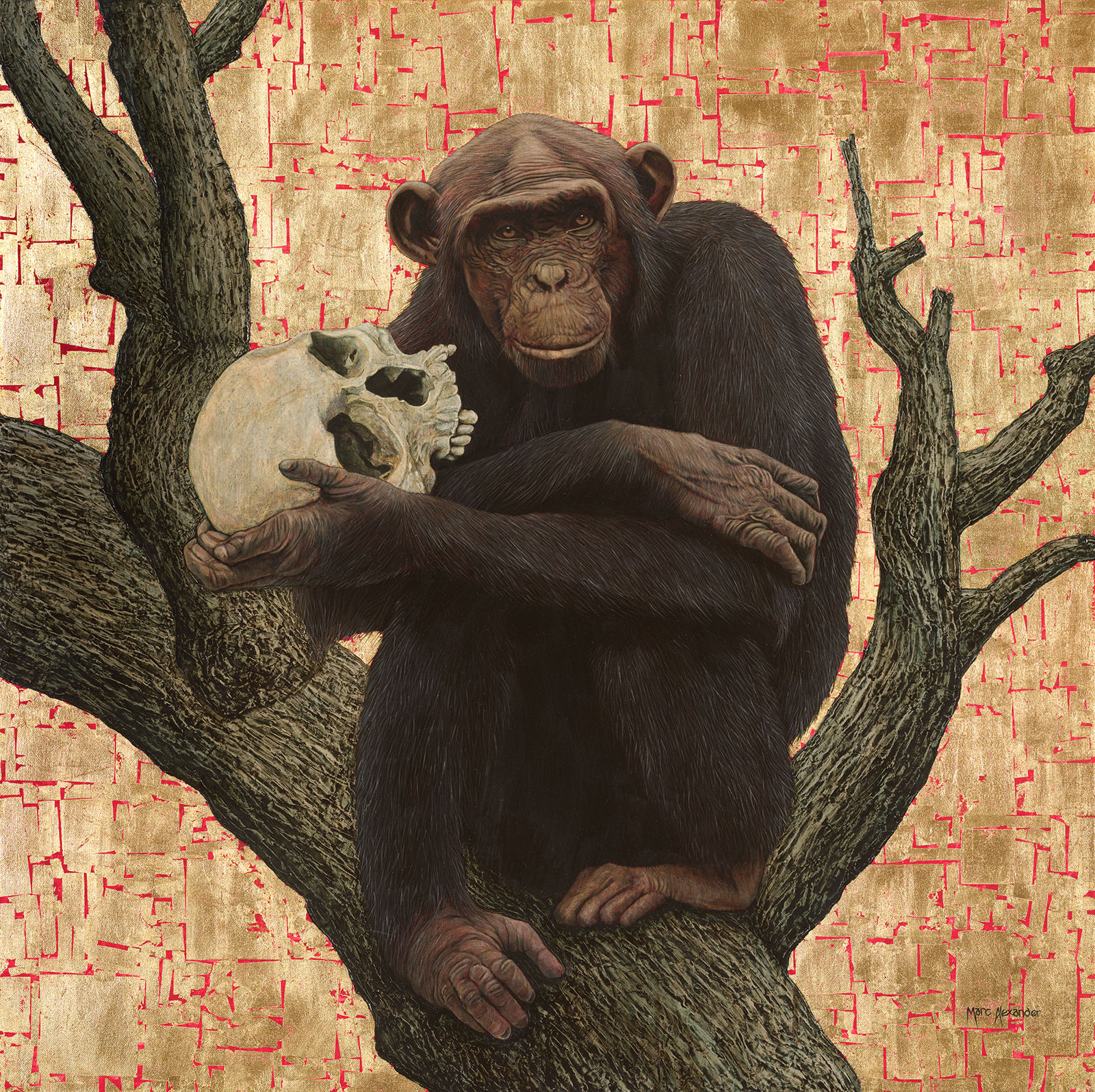##### Darwin’s Delusion
Currency conversion from USD to: [convert number=2250.00 from="usd" to="zar"] ZAR | [convert number=2250.00 from="usd" to="gbp"] GBP | [convert number=2250.00 from="usd" to="eur"] EUR     Medium: Oil and Gold leaf on Canvas Size: 100cm by 100cm SOLD
\$ 2,250.00##### Giraffe
Currency conversion from USD to: [convert number=2300.00 from="usd" to="zar"] ZAR | [convert number=2300.00 from="usd" to="gbp"] GBP | [convert number=2300.00 from="usd" to="eur"] EUR     Medium: Oil and Gold Leaf on Canvas Size: 100cm by 100cm SOLD
\$ 2,300.00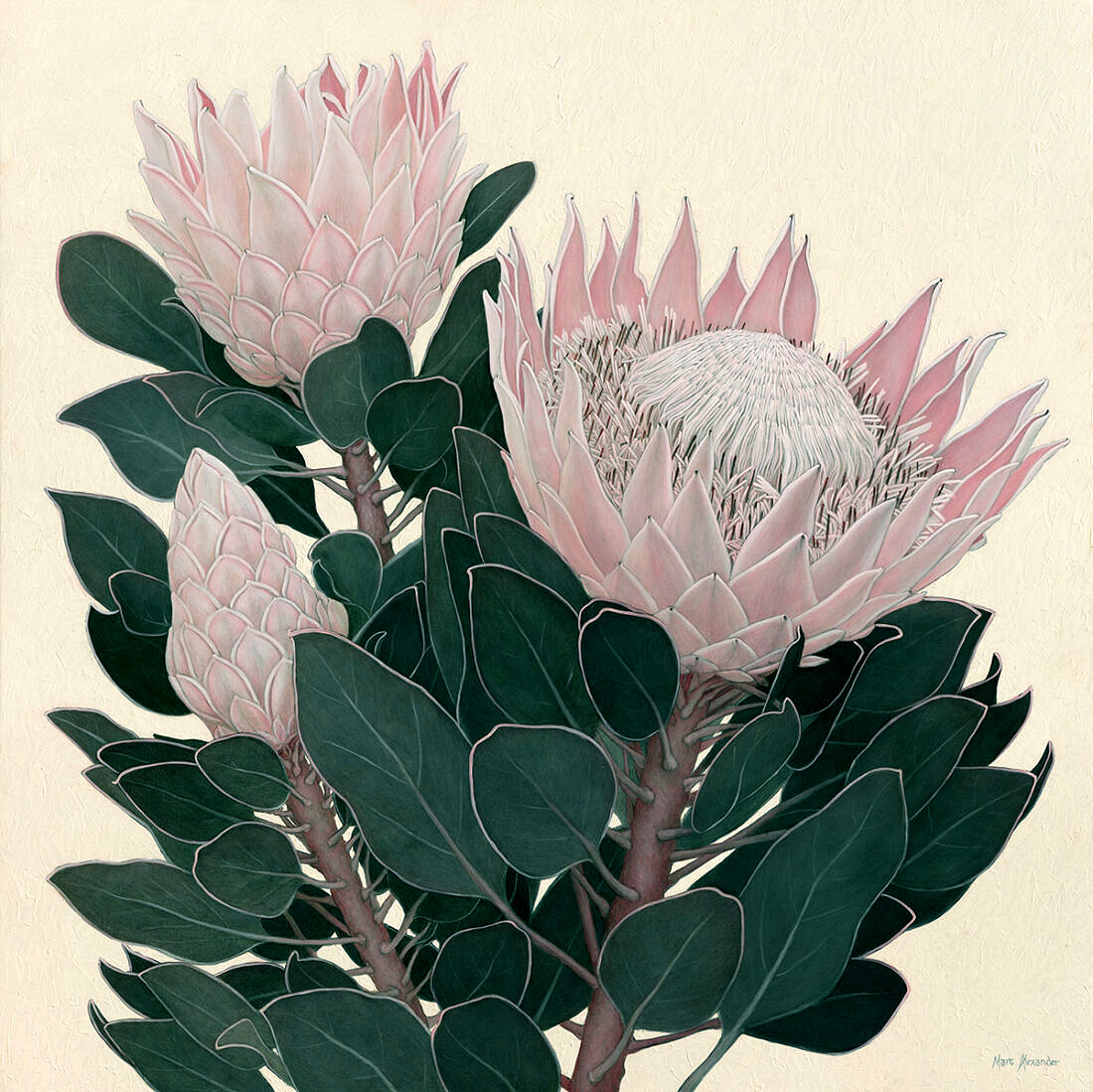##### King Protea I
Currency conversion from USD to: [convert number=2300.00 from="usd" to="zar"] ZAR | [convert number=2300.00 from="usd" to="gbp"] GBP | [convert number=2300.00 from="usd" to="eur"] EUR     Medium: Oil on Canvas Size: 100cm by 100cm SOLD
\$ 2,300.00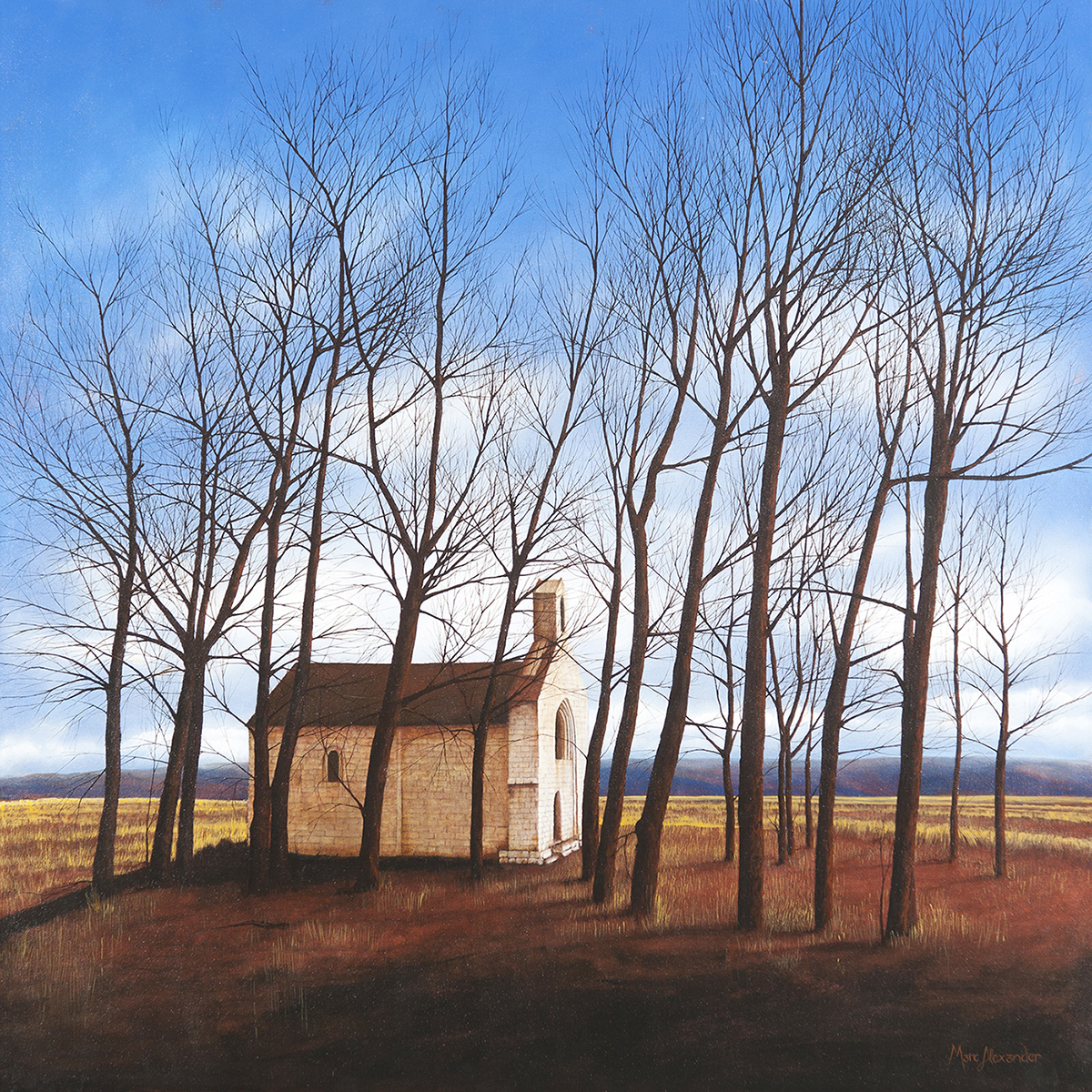##### Winter
Currency conversion from USD to: [convert number=2500.00 from="usd" to="zar"] ZAR | [convert number=2500.00 from="usd" to="gbp"] GBP | [convert number=2500.00 from="usd" to="eur"] EUR     Medium: Oil on Canvas Size: 100cm by 100cm SOLD
\$ 2,500.00##### Spring
Currency conversion from USD to: [convert number=2500.00 from="usd" to="zar"] ZAR | [convert number=2500.00 from="usd" to="gbp"] GBP | [convert number=2500.00 from="usd" to="eur"] EUR     Medium: Oil on Canvas Size: 100cm by 100cm SOLD
\$ 2,500.00##### Enchanted Forest II
Currency conversion from USD to: [convert number=2500.00 from="usd" to="zar"] ZAR | [convert number=2500.00 from="usd" to="gbp"] GBP | [convert number=2500.00 from="usd" to="eur"] EUR     Medium: Oil on Canvas Size: 100cm by 100cm SOLD
\$ 2,500.00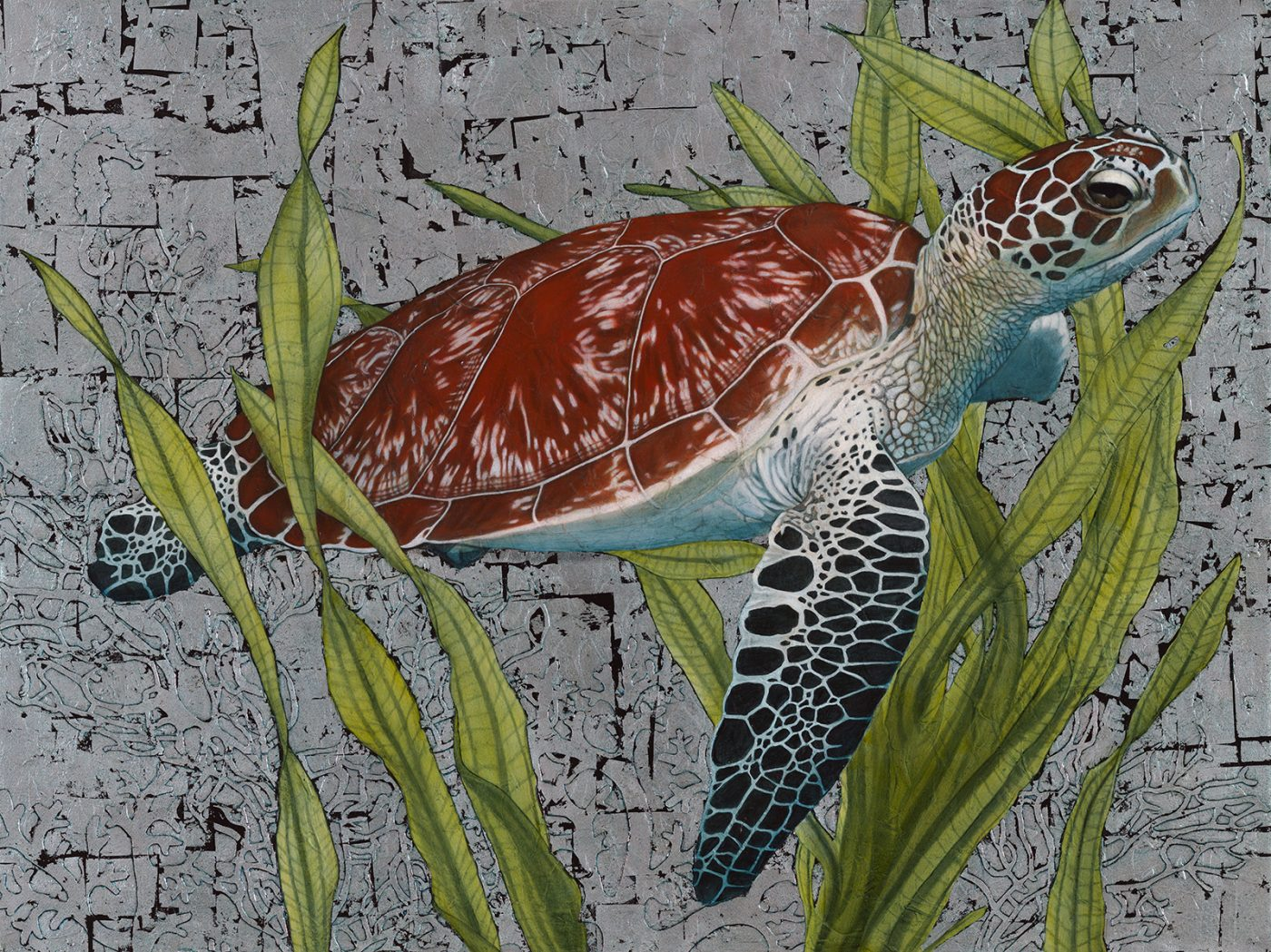##### Hawksbill Sea Turtle
Currency conversion from USD to: [convert number=2550.00 from="usd" to="zar"] ZAR | [convert number=2550.00 from="usd" to="gbp"] GBP | [convert number=2550.00 from="usd" to="eur"] EUR   Medium: Oil and Silver Leaf on Canvas Size: 90cm by 120cm SOLD
\$ 2,550.00##### Bateleur Eagle I
Currency conversion from USD to: [convert number=3450.00 from="usd" to="zar"] ZAR | [convert number=3450.00 from="usd" to="gbp"] GBP | [convert number=3450.00 from="usd" to="eur"] EUR     Medium: Oil and Gold Leaf on Canvas Size: 90cm by 120cm SOLD
\$ 3,450.00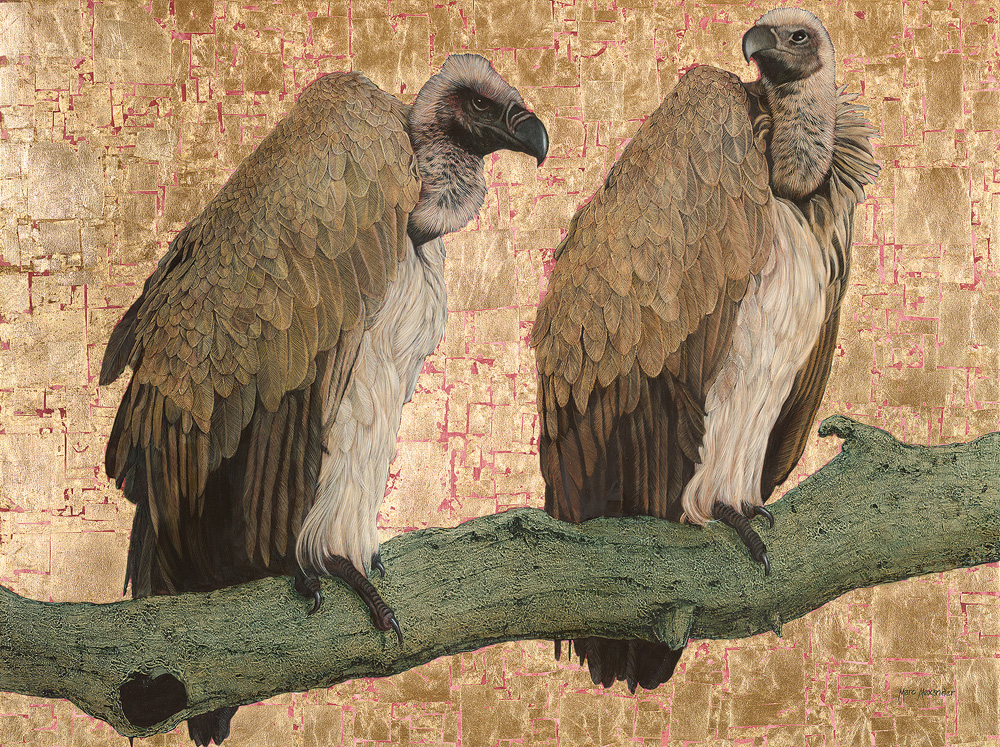##### Cape Vultures
Currency conversion from USD to: [convert number=3650.00 from="usd" to="zar"] ZAR | [convert number=3650.00 from="usd" to="gbp"] GBP | [convert number=3650.00 from="usd" to="eur"] EUR     Medium: Oil and Gold Leaf on Canvas Size: 90cm by 120cm SOLD
\$ 3,650.00##### Rhino II
Currency conversion from USD to: [convert number=3650.00 from="usd" to="zar"] ZAR | [convert number=3650.00 from="usd" to="gbp"] GBP | [convert number=3650.00 from="usd" to="eur"] EUR     Medium: Oil and Gold Leaf on Canvas Size: 90cm by 120cm SOLD
\$ 3,650.00##### Mighty Tusker
Currency conversion from USD to: [convert number=3650.00 from="usd" to="zar"] ZAR | [convert number=3650.00 from="usd" to="gbp"] GBP | [convert number=3650.00 from="usd" to="eur"] EUR     Medium: Oil and Gold Leaf on Canvas Size: 90cm by 120cm SOLD
\$ 3,650.00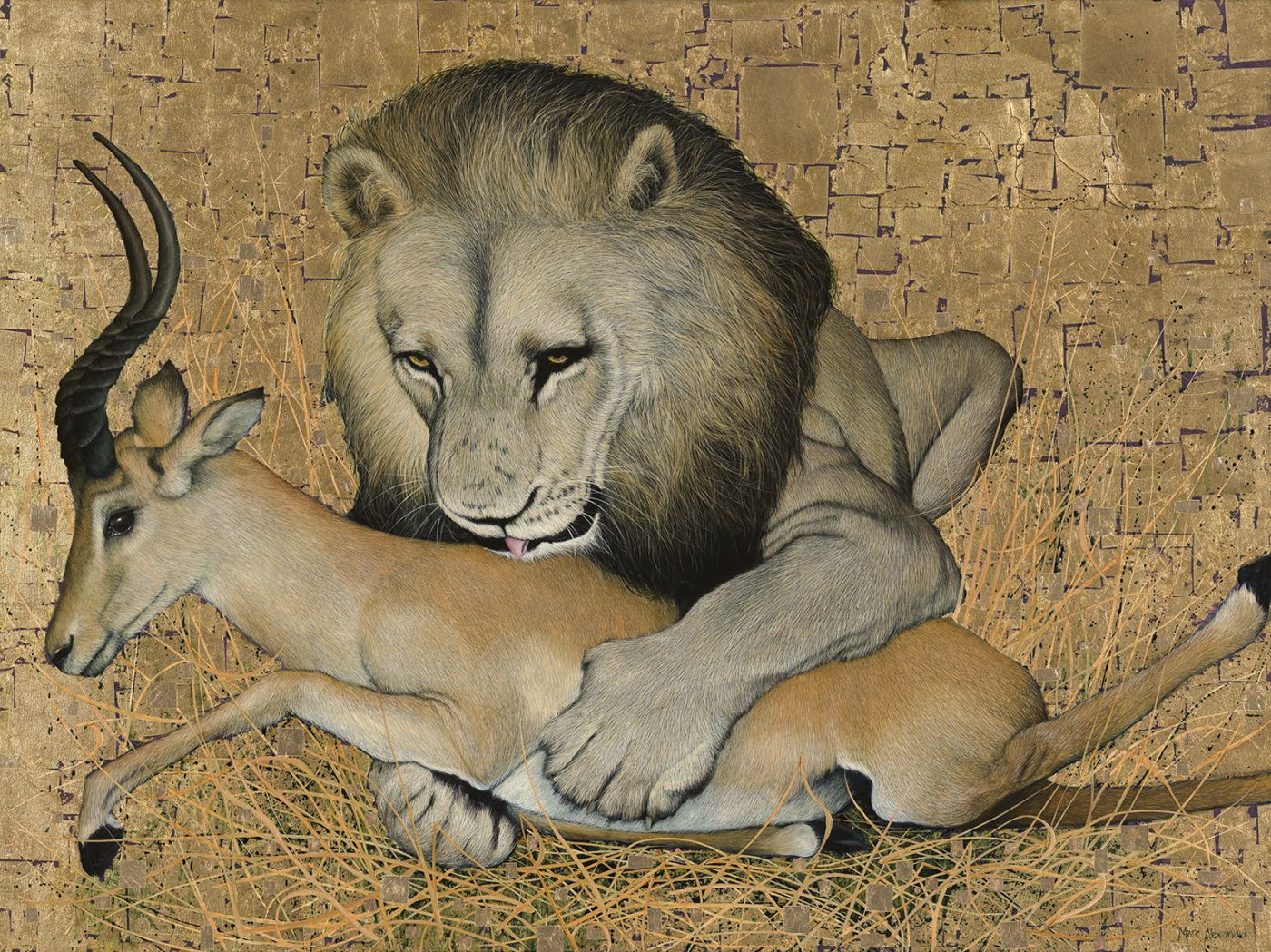##### The Kill
Currency conversion from USD to: [convert number=3650.00 from="usd" to="zar"] ZAR | [convert number=3650.00 from="usd" to="gbp"] GBP | [convert number=3650.00 from="usd" to="eur"] EUR     Medium: Oil and Gold Leaf on Canvas Size: 90cm by 120cm SOLD
\$ 3,650.00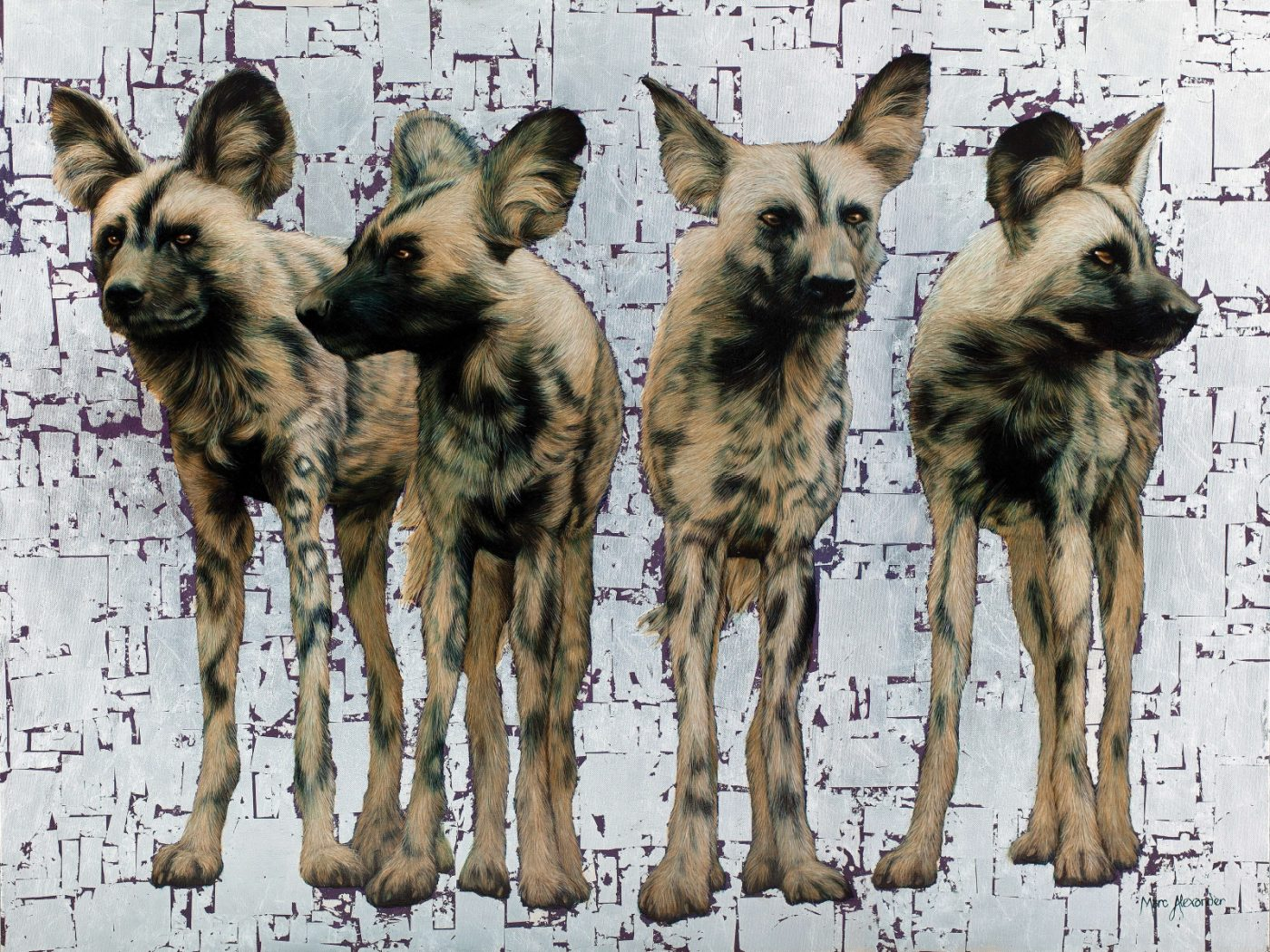##### Wild Dogs Pack of 4
Currency conversion from USD to: [convert number=3650.00 from="usd" to="zar"] ZAR | [convert number=3650.00 from="usd" to="gbp"] GBP | [convert number=3650.00 from="usd" to="eur"] EUR     Medium: Oil and Silver Leaf on Canvas Size: 90cm by 120cm SOLD
\$ 3,650.00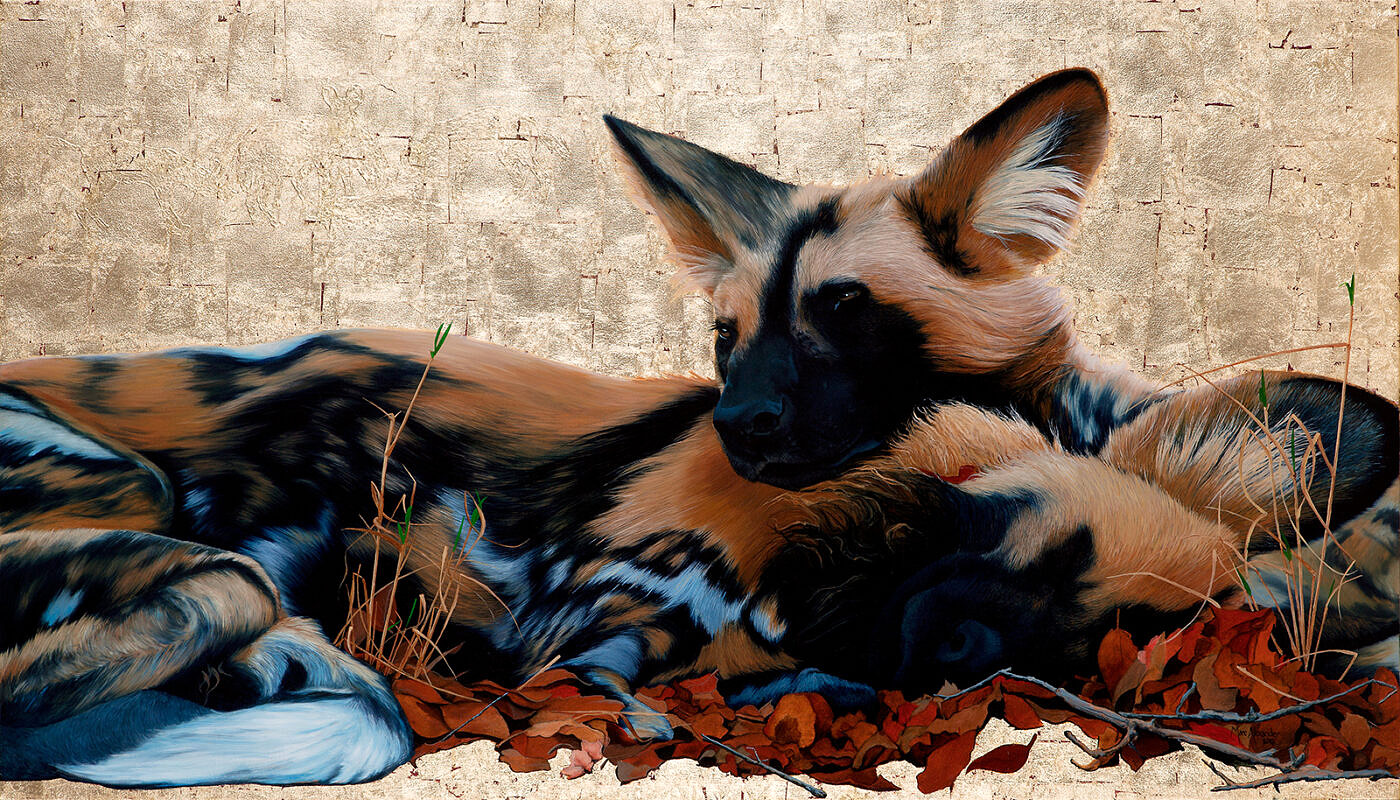##### Wild Dogs I
Currency conversion from USD to: [convert number=3650.00 from="usd" to="zar"] ZAR | [convert number=3650.00 from="usd" to="gbp"] GBP | [convert number=3650.00 from="usd" to="eur"] EUR     Medium: Oil and Gold Leaf on Canvas Size: 80cm by 140cm SOLD
\$ 3,650.00##### Wild Dogs at Dawn
Currency conversion from USD to: [convert number=3650.00 from="usd" to="zar"] ZAR | [convert number=3650.00 from="usd" to="gbp"] GBP | [convert number=3650.00 from="usd" to="eur"] EUR     Medium: Oil and Gold Leaf on Canvas Size: 80cm by 140cm SOLD
\$ 3,650.00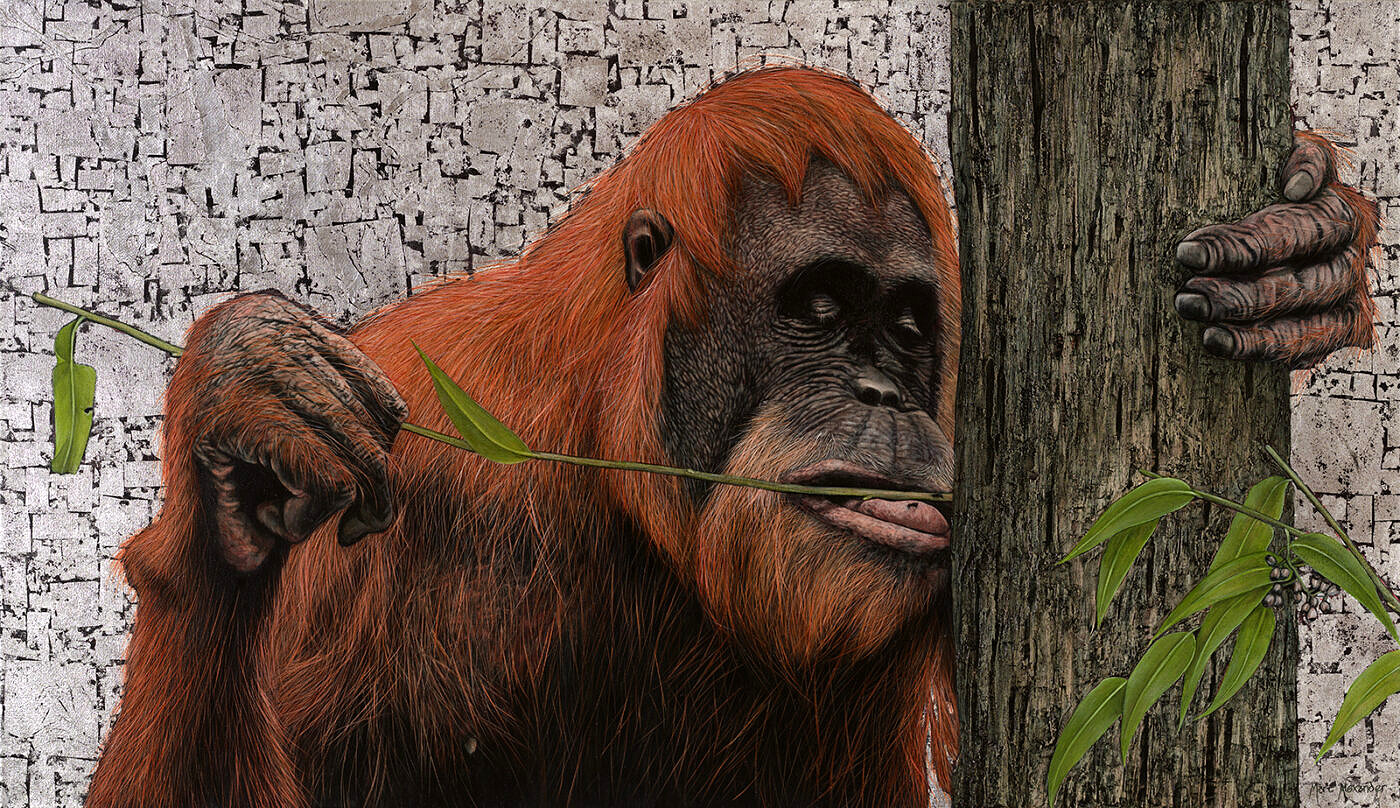##### Sumatran Orangutan
Currency conversion from USD to: [convert number=3650.00 from="usd" to="zar"] ZAR | [convert number=3650.00 from="usd" to="gbp"] GBP | [convert number=3650.00 from="usd" to="eur"] EUR     Medium: Oil and Silver Leaf on Canvas Size: 80cm by 140cm SOLD
\$ 3,650.00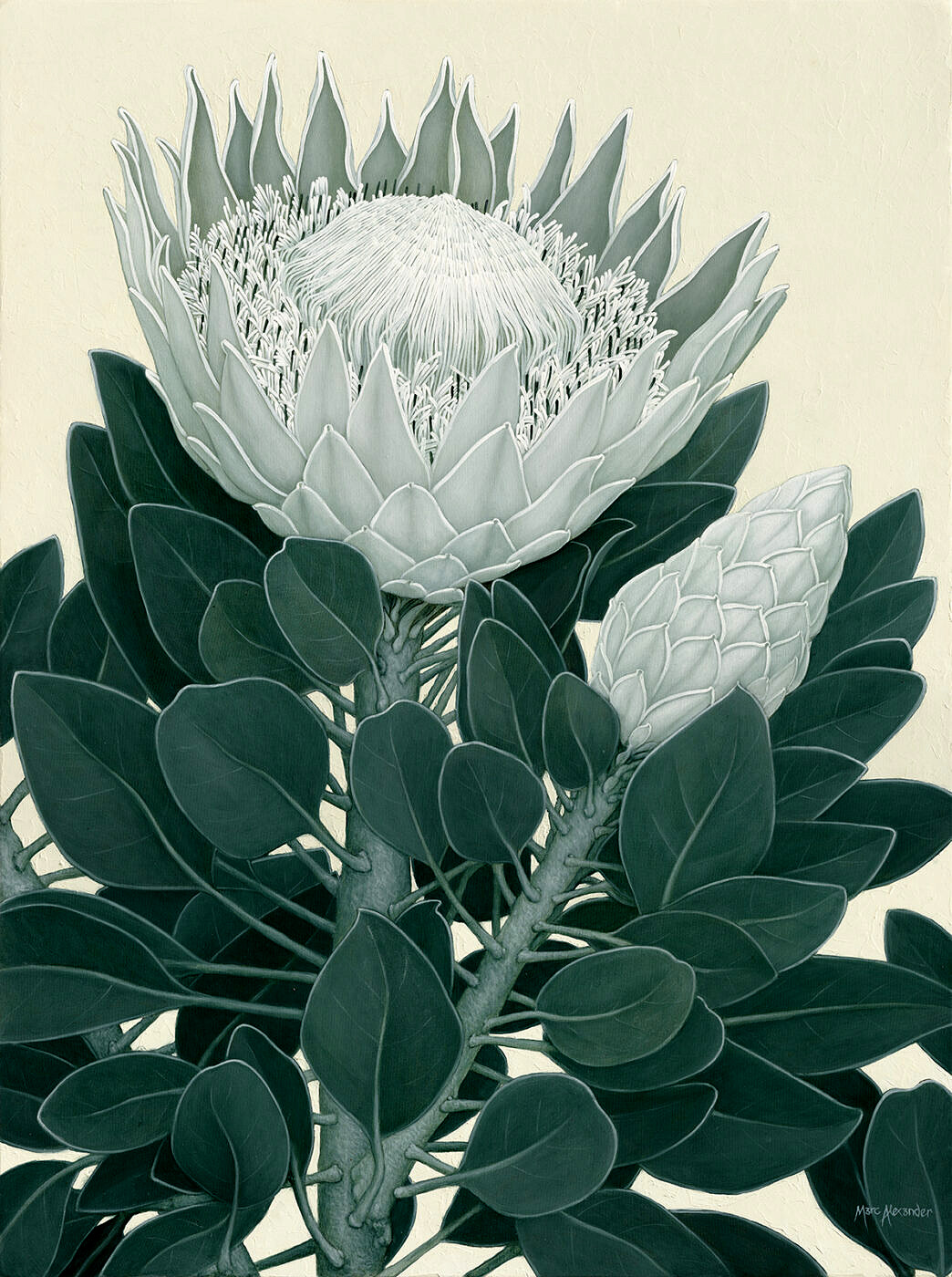##### King Protea II
Currency conversion from USD to: [convert number=3650.00 from="usd" to="zar"] ZAR | [convert number=3650.00 from="usd" to="gbp"] GBP | [convert number=3650.00 from="usd" to="eur"] EUR     Medium: Oil on Canvas Size: 1200cm by 90cm SOLD
\$ 3,650.00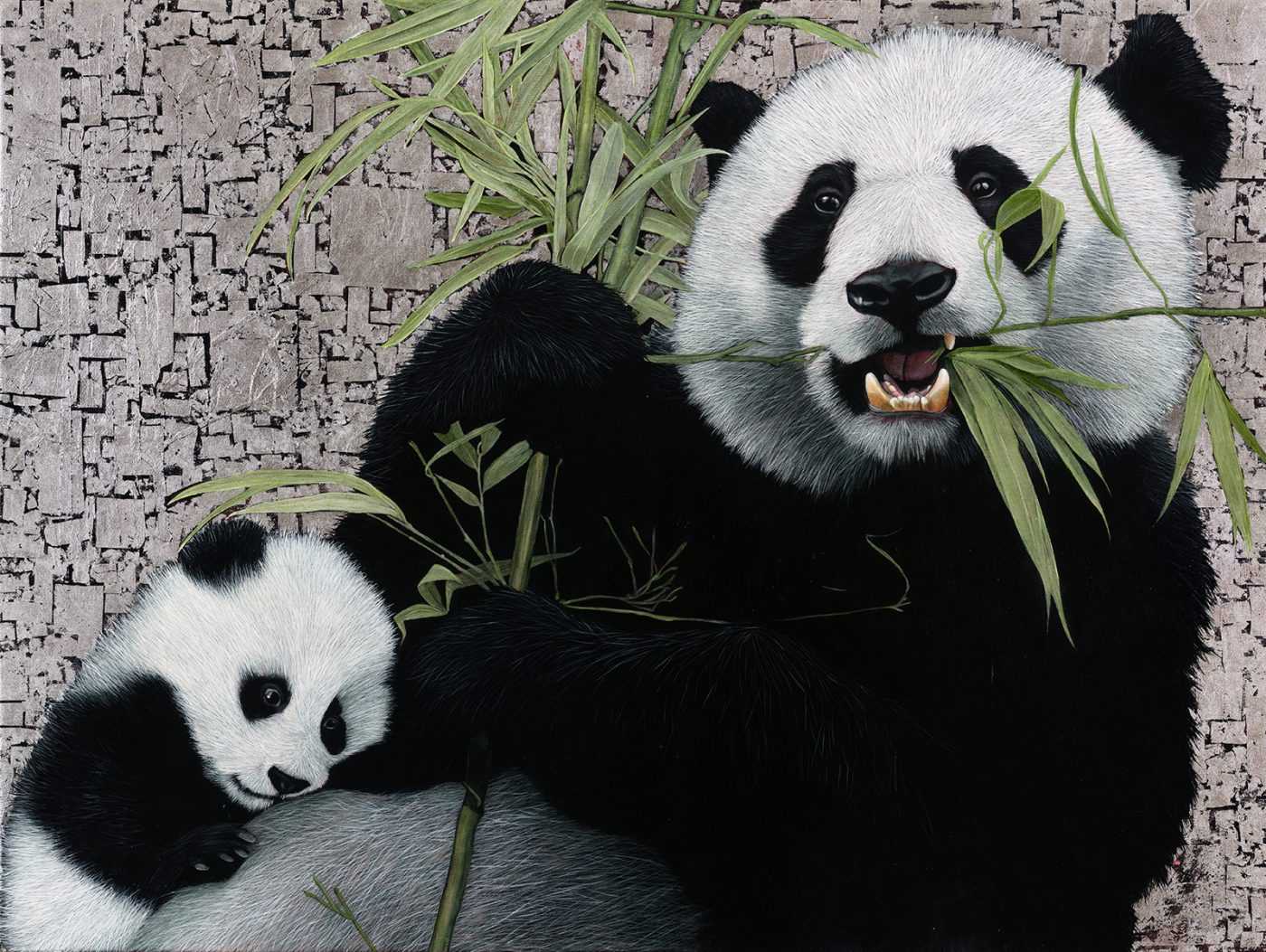##### Giant Pandas
Currency conversion from USD to: [convert number=3700.00 from="usd" to="zar"] ZAR | [convert number=3700.00 from="usd" to="gbp"] GBP | [convert number=3700.00 from="usd" to="eur"] EUR   Medium: Oil and Silver Leaf on Canvas Size: 90cm by 120cm SOLD
\$ 3,700.00##### Bateleur Eagle II
Currency conversion from USD to: [convert number=3850.00 from="usd" to="zar"] ZAR | [convert number=3850.00 from="usd" to="gbp"] GBP | [convert number=3850.00 from="usd" to="eur"] EUR     Medium: Oil and Gold Leaf on Canvas Size: 120cm by 90cm SOLD
\$ 3,850.00##### African Buffalo
Currency conversion from USD to: [convert number=3450.00 from="usd" to="zar"] ZAR | [convert number=3450.00 from="usd" to="gbp"] GBP | [convert number=3450.00 from="usd" to="eur"] EUR     Medium: Oil and Gold Leaf on Canvas Size: 90cm by 120cm SOLD
\$ 3,975.00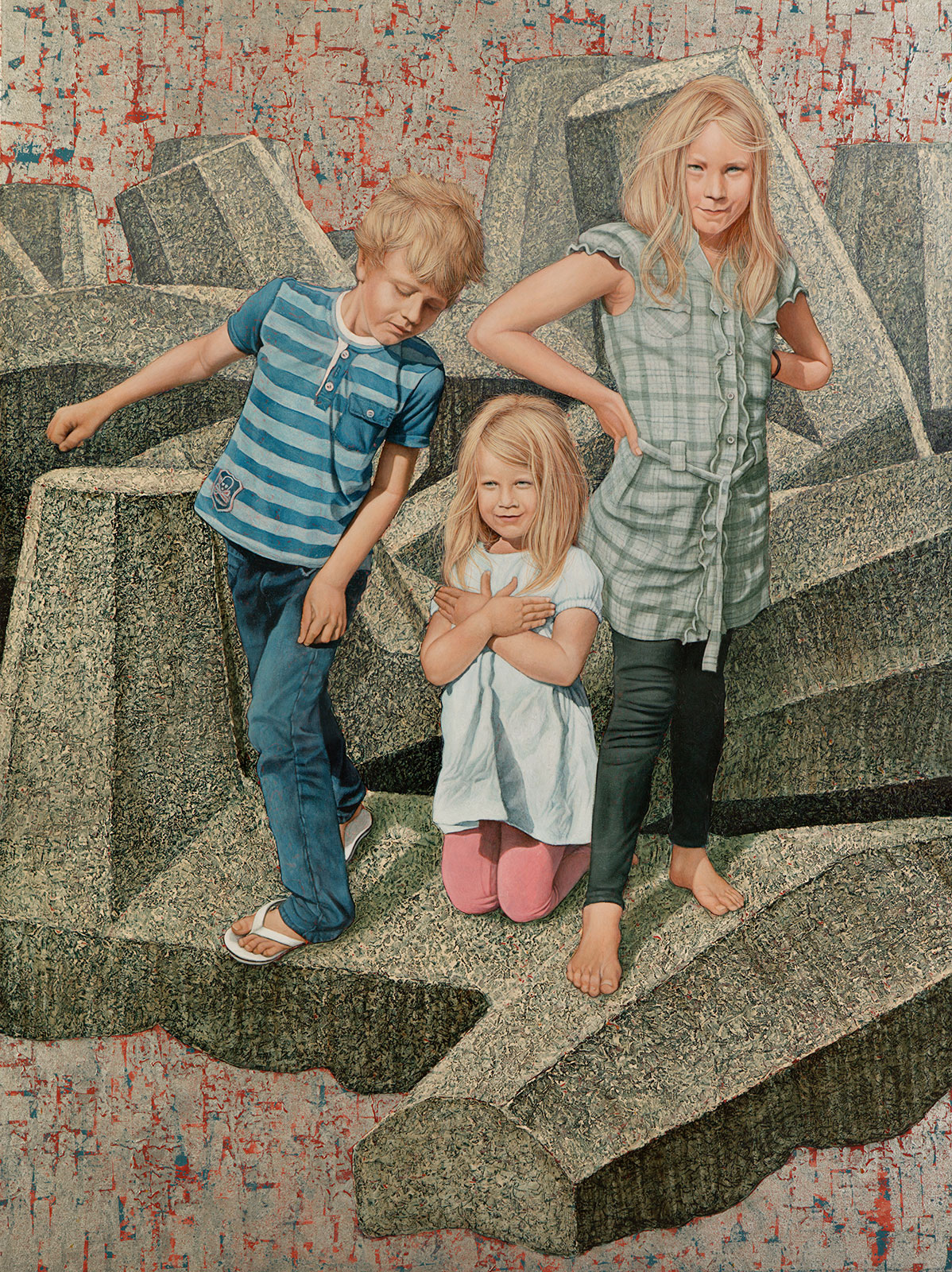##### Child’s Play
Currency conversion from USD to: [convert number=4500.00 from="usd" to="zar"] ZAR | [convert number=4500.00 from="usd" to="gbp"] GBP | [convert number=4500.00 from="usd" to="eur"] EUR     Medium: Oil and Silver Leaf on Canvas Size: 120cm by 90cm
\$ 4,500.00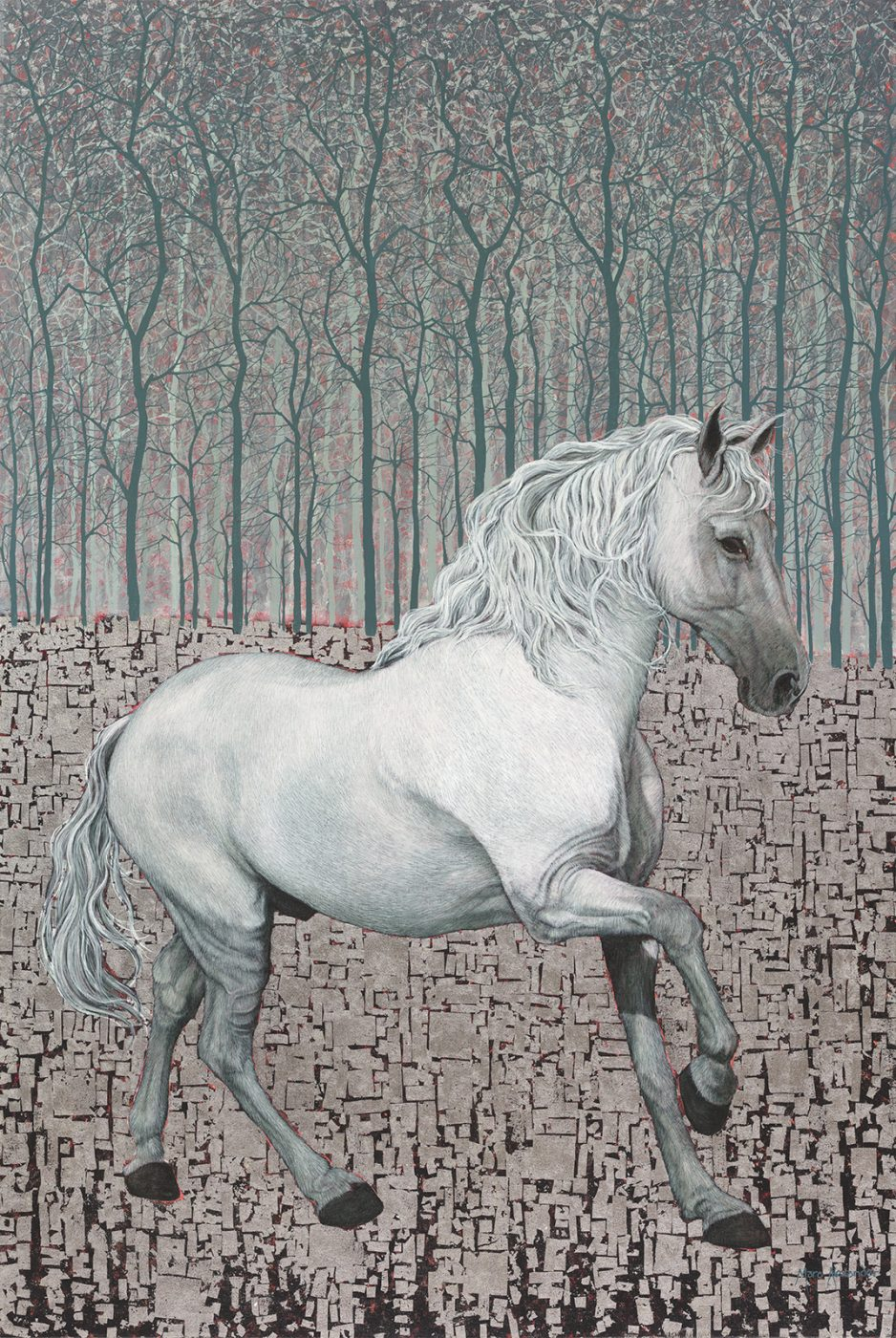Currency conversion from USD to: [convert number=4600.00 from="usd" to="zar"] ZAR | [convert number=4600.00 from="usd" to="gbp"] GBP | [convert number=4600.00 from="usd" to="eur"] EUR   Medium: Oil and Silver Leaf on Canvas Size: 100cm by 150cm SOLD
\$ 4,600.00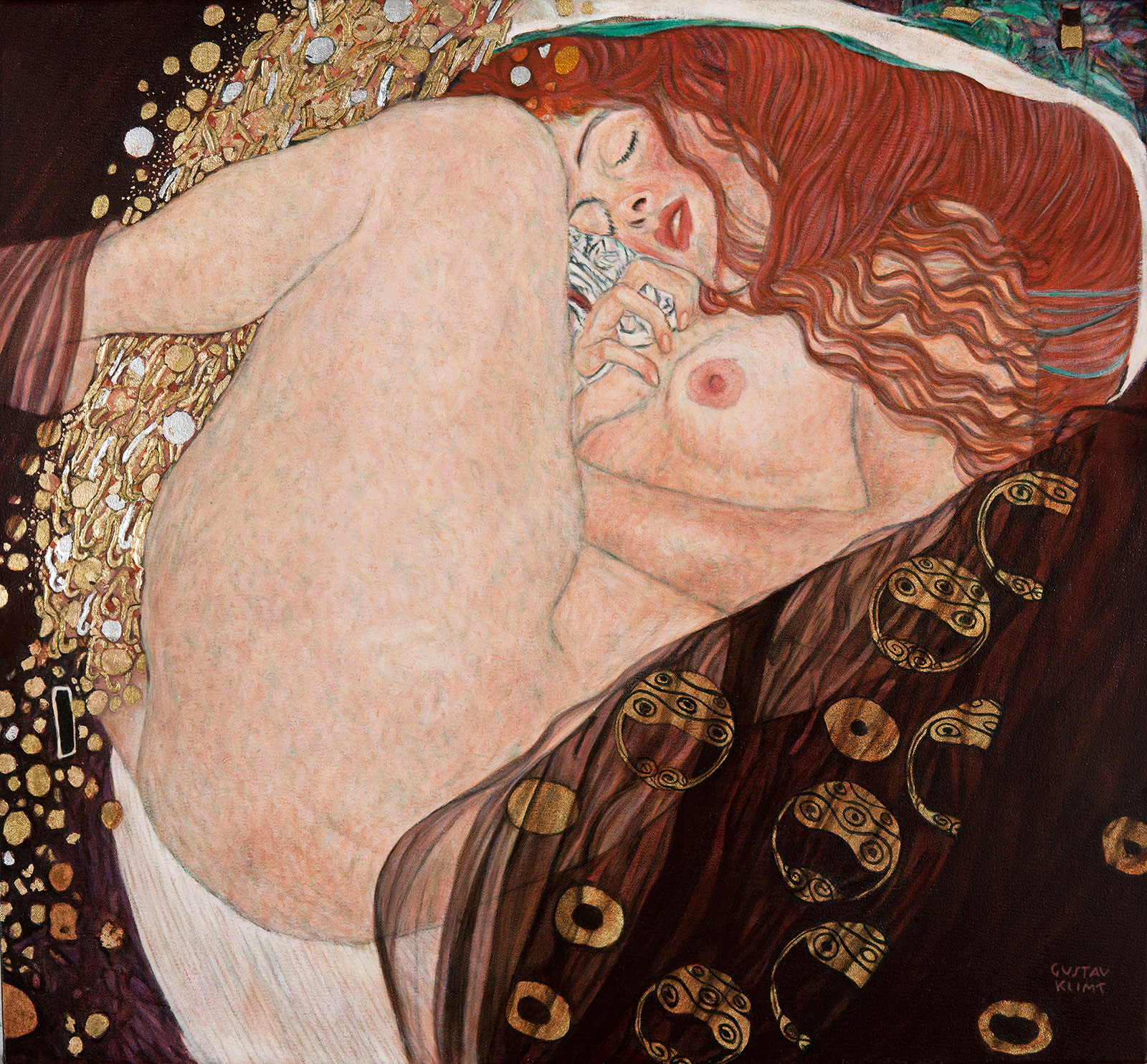##### Danaë After Klimt
Currency conversion from USD to: [convert number=4850.00 from="usd" to="zar"] ZAR | [convert number=4850.00 from="usd" to="gbp"] GBP | [convert number=4850.00 from="usd" to="eur"] EUR     Medium: Oil and Gold Leaf on Canvas Size: 77cm by 83cm Click here to order a Reproduction
\$ 4,850.00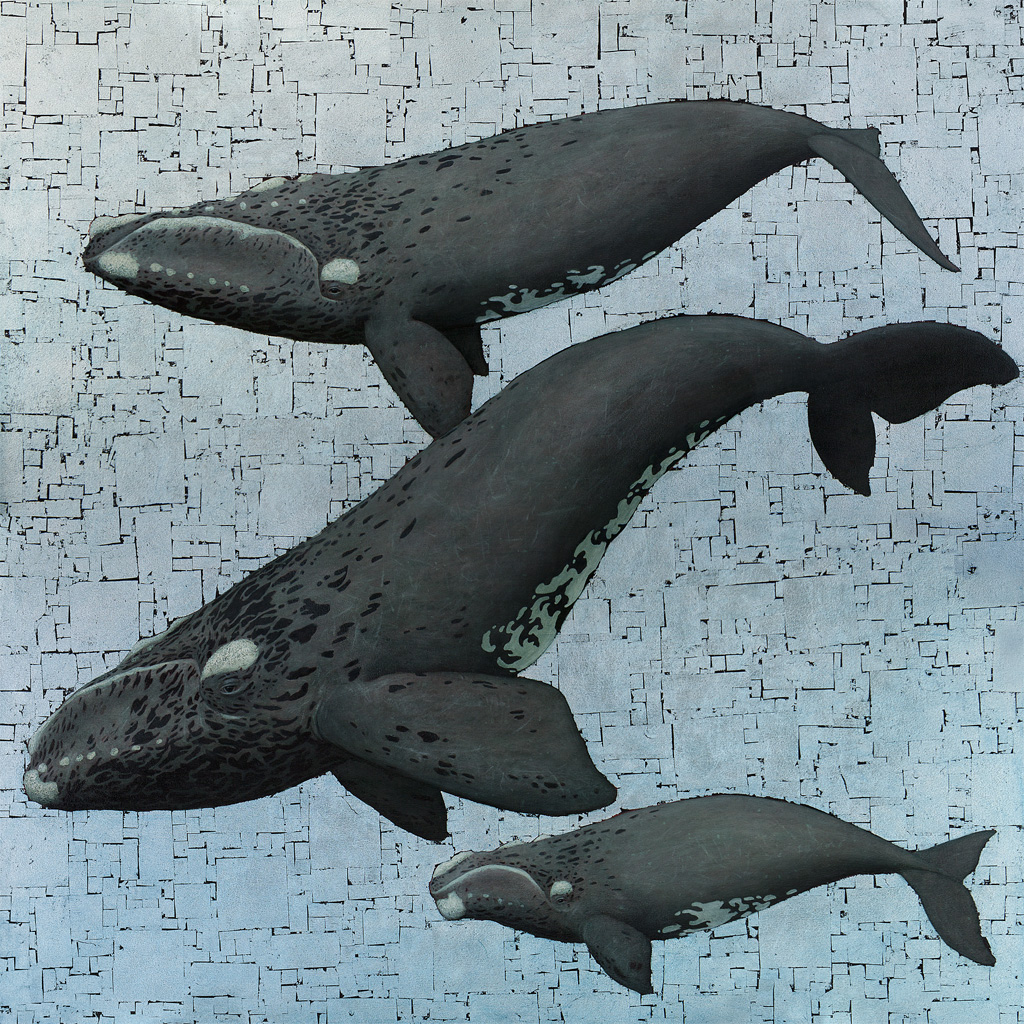##### Whale Season
Currency conversion from USD to: [convert number=7250.00 from="usd" to="zar"] ZAR | [convert number=7250.00 from="usd" to="gbp"] GBP | [convert number=7250.00 from="usd" to="eur"] EUR     Medium: Oil and Silver leaf on Canvas Size: 138cm by 138cm SOLD
\$ 7,250.00##### The Kiss after Klimt
Currency conversion from USD to: [convert number=8150.00 from="usd" to="zar"] ZAR | [convert number=8150.00 from="usd" to="gbp"] GBP | [convert number=8150.00 from="usd" to="eur"] EUR     Medium: Oil and Gold Leaf on Canvas Size: 180cm by 180cm Click here to order a Reproduction
\$ 8,150.00##### Snow Leopard II
Currency conversion from USD to: [convert number=10050.00 from="usd" to="zar"] ZAR | [convert number=10050.00 from="usd" to="gbp"] GBP | [convert number=10050.00 from="usd" to="eur"] EUR     Medium: Oil on Canvas Size: 120cm by 200cm SOLD
\$ 10,050.00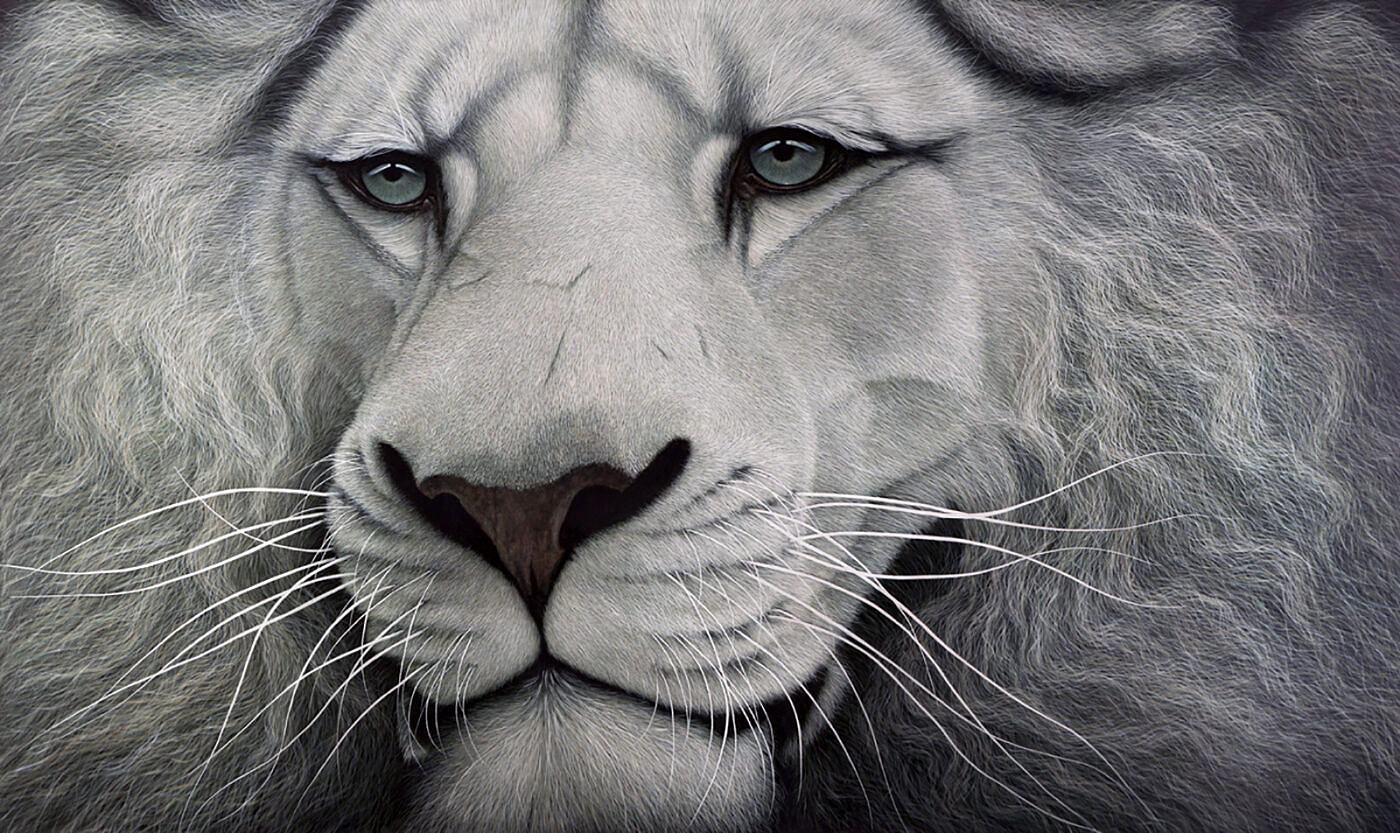##### White Lion
Currency conversion from USD to: [convert number=11000.00 from="usd" to="zar"] ZAR | [convert number=11000.00 from="usd" to="gbp"] GBP | [convert number=11000.00 from="usd" to="eur"] EUR     Medium: Oil on Canvas Size: 120cm by 200cm SOLD
\$ 11,000.00